WEST Math: Solving Trigonometric Equations Chapter Exam

Exam Instructions:

Choose your answers to the questions and click 'Next' to see the next set of questions. You can skip questions if you would like and come back to them later with the yellow "Go To First Skipped Question" button. When you have completed the practice exam, a green submit button will appear. Click it to see your results. Good luck!

Page 1

Question 2 2. Solve the following trigonometric equation for x.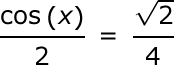Page 3

Question 13 13. Solve for the value of x in the trigonometric equation below. (Use the domain -π/2 to π/2)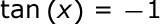Question 14 14. Solve the following equation for θ.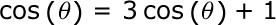Page 5

Question 23 23. Solve the following trigonometric equation for θ.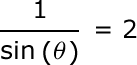Question 25 25. Solve the following equation for x.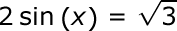WEST Math: Solving Trigonometric Equations Chapter Exam Instructions

Choose your answers to the questions and click 'Next' to see the next set of questions. You can skip questions if you would like and come back to them later with the yellow "Go To First Skipped Question" button. When you have completed the practice exam, a green submit button will appear. Click it to see your results. Good luck!

Support